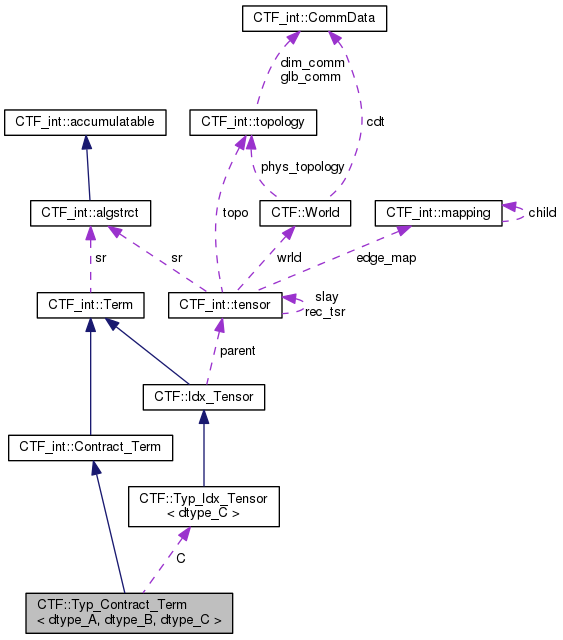Cyclops Tensor Framework parallel arithmetic on multidimensional arrays
CTF::Typ_Contract_Term< dtype_A, dtype_B, dtype_C > Class Template Reference

`#include <idx_tensor.h>`

Inheritance diagram for CTF::Typ_Contract_Term< dtype_A, dtype_B, dtype_C >:[legend]
Collaboration diagram for CTF::Typ_Contract_Term< dtype_A, dtype_B, dtype_C >:[legend]

## Public Member Functions

Typ_Contract_Term (Typ_Idx_Tensor< dtype_C > *C_, Typ_Sum_Term< dtype_A, dtype_B > S)

void operator, (std::function< dtype_C(dtype_A, dtype_B)> f)

void operator, (std::function< void(dtype_A, dtype_B, dtype_C &)> f)Public Member Functions inherited from CTF_int::Contract_Term
Contract_Term (Term *B, Term *A)
creates sum term for B+A More...

~Contract_Term ()

Contract_Term (Contract_Term const &other, std::map< tensor *, tensor * > *remap=NULL)

Termclone (std::map< tensor *, tensor * > *remap=NULL) const
base classes must implement this copy function to retrieve pointer More...

void execute (CTF::Idx_Tensor output) const
override execution to to contract operands and add them to output More...

std::vector< char > get_uniq_inds () const
find list of unique indices that are involved in this term More...

void get_inputs (std::set< CTF::Idx_Tensor *, tensor_name_less > *inputs_set) const
appends the tensors this depends on to the input set More...

CTF::Idx_Tensor execute (std::vector< char > out_inds) const
evalues the expression to produce an intermediate with all expression indices remaining More...

double estimate_time (CTF::Idx_Tensor output) const
estimates the cost of a contract term More...

CTF::Idx_Tensor estimate_time (double &cost, std::vector< char > out_inds) const
estimates the cost the expression to produce an intermediate with all expression indices remaining More...

Contract_Term operator* (Term const &A) const
override contraction to grow vector rather than create recursive terms More...

CTF::Worldwhere_am_i () const
negates term More...Public Member Functions inherited from CTF_int::Term
Term (algstrct const *sr)

virtual ~Term ()

void mult_scl (char const *mulscl)
multiply scaling factor by mulscl More...

Contract_Term operator* (Term const &A) const
constructs a new term which multiplies by tensor A More...

Contract_Term operator* (int64_t scl) const
multiples by a constant More...

Contract_Term operator* (double scl) const

Sum_Term operator+ (Term const &A) const
constructs a new term by addition of two terms More...

Sum_Term operator+ (double scl) const

Sum_Term operator+ (int64_t scl) const

Sum_Term operator- (Term const &A) const
constructs a new term by subtracting term A More...

Sum_Term operator- (double scl) const

Sum_Term operator- (int64_t scl) const

Termoperator- ()

void operator= (CTF::Idx_Tensor const &B)
A = B, compute any operations on operand B and set. More...

void operator= (Term const &B)

void operator+= (Term const &B)

void operator-= (Term const &B)

void operator*= (Term const &B)

void operator= (double scl)

void operator+= (double scl)

void operator<< (CTF_int::Term const &B)

void operator<< (double scl)

void operator-= (double scl)

void operator*= (double scl)

void operator= (int64_t scl)

void operator+= (int64_t scl)

void operator-= (int64_t scl)

void operator*= (int64_t scl)

void operator= (int scl)

void operator+= (int scl)

void operator-= (int scl)

void operator*= (int scl)

operator float () const
cast to float (works only if tensor type is castable to float) allows a scalar output More...

operator double () const
cast to double (works only if tensor type is castable to double) allows a scalar output More...

operator int64_t () const
cast to int64_t (works only if tensor type is castable to int64_t) allows a scalar output More...

operator int () const
cast to int64_t (works only if tensor type is castable to int64_t) allows a scalar output More...

## Data Fields

Typ_Idx_Tensor< dtype_C > * CData Fields inherited from CTF_int::Contract_Term
std::vector< Term * > operandsData Fields inherited from CTF_int::Term
char * scale

algstrctsr

## Detailed Description

### template<typename dtype_A, typename dtype_B, typename dtype_C> class CTF::Typ_Contract_Term< dtype_A, dtype_B, dtype_C >

Definition at line 171 of file idx_tensor.h.

## Constructor & Destructor Documentation

template<typename dtype_A, typename dtype_B, typename dtype_C>
 CTF::Typ_Contract_Term< dtype_A, dtype_B, dtype_C >::Typ_Contract_Term ( Typ_Idx_Tensor< dtype_C > * C_, Typ_Sum_Term< dtype_A, dtype_B > S )
inline

Definition at line 206 of file idx_tensor.h.

## Member Function Documentation

template<typename dtype_A, typename dtype_B, typename dtype_C>
 void CTF::Typ_Contract_Term< dtype_A, dtype_B, dtype_C >::operator, ( std::function< dtype_C(dtype_A, dtype_B)> f )
inline

Definition at line 213 of file idx_tensor.h.

template<typename dtype_A, typename dtype_B, typename dtype_C>
 void CTF::Typ_Contract_Term< dtype_A, dtype_B, dtype_C >::operator, ( std::function< void(dtype_A, dtype_B, dtype_C &)> f )
inline

Definition at line 217 of file idx_tensor.h.

## Field Documentation

template<typename dtype_A, typename dtype_B, typename dtype_C>
 Typ_Idx_Tensor* CTF::Typ_Contract_Term< dtype_A, dtype_B, dtype_C >::C

Definition at line 205 of file idx_tensor.h.

The documentation for this class was generated from the following file: